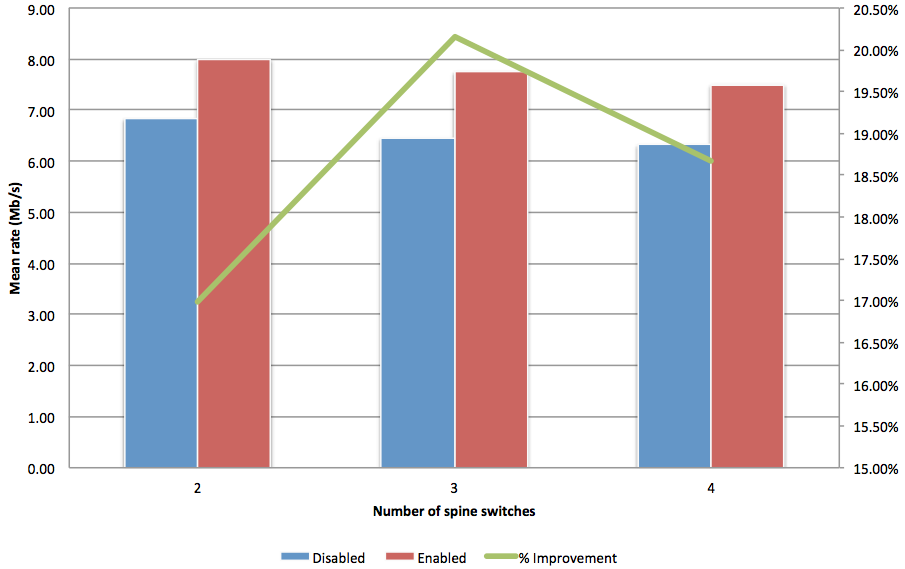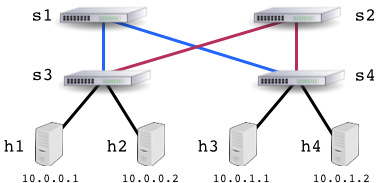## Tuesday, May 13, 2014

### Load balancing large flows on multi-path networksFigure 1: Active control of large flows in a multi-path topology
Figure 1 shows initial results from the Mininet integrated hybrid OpenFlow testbed demonstrating that active steering of large flows using a performance aware SDN controller significantly improves network throughput of multi-path network topologies.Figure 2: Two path topology
The graph in Figure 1 summarizes results from topologies with 2, 3 and 4 equal cost paths. For example, the Mininet topology in Figure 2 has two equal cost paths of 10Mbit/s (shown in blue and red). The iperf traffic generator was used to create a continuous stream of 20 second flows from h1 to h3 and from h2 to h4. If traffic were perfectly balanced, each flow would achieve 10Mbit/s throughput. However, Figure 1 shows that the throughput obtained using hash based ECMP load balancing is approximately 6.8Mbit/s. Interestingly, the average link throughput decreases as additional paths are added, dropping to approximately 6.2Mbit/s with four equal cost paths (see the blue bars in Figure 1).

To ensure that packets in a flow arrive in order at their destination, switch s3 computes a hash function over selected fields in the packets (e.g. source and destination IP addresses + source and destination TCP ports) and picks a link based on the value of the hash, e.g.
```index = hash(packet fields) % linkgroup.sizeFigure 3: Performance optimizing hybrid OpenFlow controller